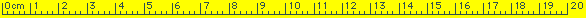The answer is ...17 human hands = 170 centimetersThe answer is ...12 human hands = 120 centimetersThe answer is ...8 human hands = 80 centimetersThe answer is ...10 human hands = 100 centimetersThe answer is ...15 human hands = 150 centimetersThe answer is ...9 human hands = 90 centimetersThe answer is ...14 human hands = 140 centimetersThe answer is ...18 human hands = 180 centimetersThe answer is ...11 human hands = 110 centimetersThe answer is ...16 human hands = 160 centimeters. Home Page